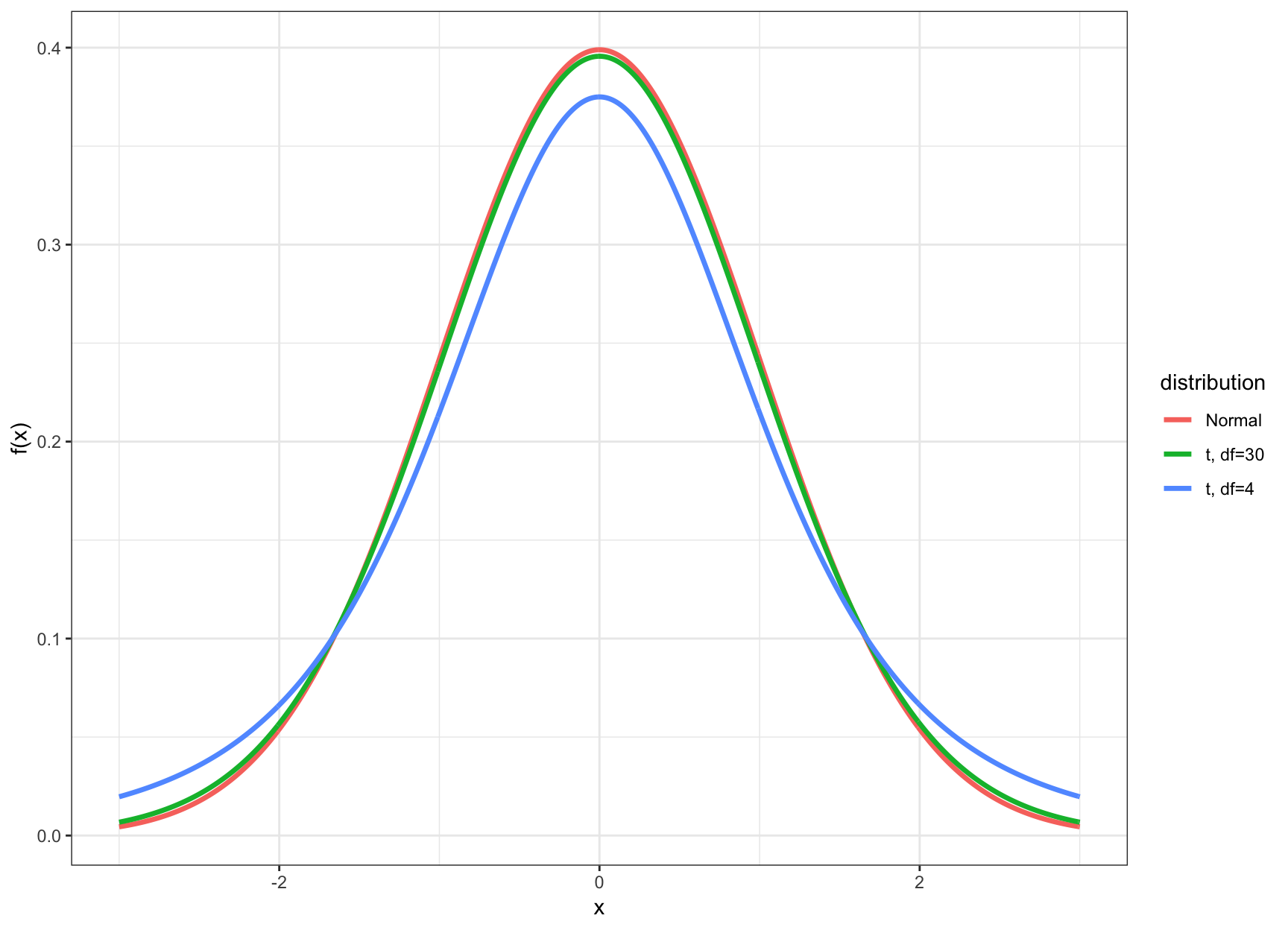29 The t Distribution

29.1 Normal, Unknown Variance

Suppose a sample of $$n$$ data points is modeled by $$X_1, X_2, \ldots, X_n \stackrel{{\rm iid}}{\sim} \mbox{Normal}(\mu, \sigma^2)$$ where $$\sigma^2$$ is unknown. Recall that $$S = \sqrt{\frac{\sum_{i=1}^n (X_i - \overline{X})^2}{n-1}}$$ is the sample standard deviation.

The statistic $\frac{\overline{X} - \mu}{S/\sqrt{n}}$

has a $$t_{n-1}$$ distribution, a t-distribution with $$n-1$$ degrees of freedom.

29.2 Aside: Chi-Square Distribution

Suppose $$Z_1, Z_2, \ldots, Z_v \stackrel{{\rm iid}}{\sim} \mbox{Normal}(0, 1)$$. Then $$Z_1^2 + Z_2^2 + \cdots + Z_v^2$$ has a $$\chi^2_v$$ distribution, where $$v$$ is the degrees of freedom.

This $$\chi^2_v$$ rv has a pdf, expected value equal to $$v$$, and variance equal to $$2v$$.

Also,

$\frac{(n-1) S^2}{\sigma^2} \sim \chi^2_{n-1}.$

29.3 Theoretical Basis of the t

Suppose that $$Z \sim \mbox{Normal}(0,1)$$, $$X \sim \chi^2_v$$, and $$Z$$ and $$X$$ are independent. Then $$\frac{Z}{\sqrt{X/v}}$$ has a $$t_v$$ distribution.

Since $$\frac{\overline{X} - \mu}{\sigma/\sqrt{n}} \sim \mbox{Normal}(0,1)$$ and $$\overline{X}$$ and $$S^2$$ are independent (shown later), it follows that the following has a $$t_{n-1}$$ distribution:

$\frac{\overline{X} - \mu}{S/\sqrt{n}}.$

29.4 When Is t Utilized?

• The t distribution and its corresponding CI’s and HT’s are utilized when the data are Normal (or approximately Normal) and $$n$$ is small
• Small typically means that $$n < 30$$
• In this case the inference based on the t distribution will be more accurate
• When $$n \geq 30$$, there is very little difference between using $$t$$-statistics and $$z$$-statistics

29.5t vs Normal29.6t Percentiles

We calculated percentiles of the Normal(0,1) distribution (e.g., $$z_\alpha$$). We can do the analogous calculation with the t distribution.

Let $$t_\alpha$$ be the $$\alpha$$ percentile of the t distribution. Examples:

> qt(0.025, df=4) # alpha = 0.025
 -2.776445
> qt(0.05, df=4)
 -2.131847
> qt(0.95, df=4)
 2.131847
> qt(0.975, df=4)
 2.776445

29.7 Confidence Intervals

Here is a $$(1-\alpha)$$-level CI for $$\mu$$ using this distribution:

$\left(\hat{\mu} - |t_{\alpha/2}| \frac{s}{\sqrt{n}}, \hat{\mu} + |t_{\alpha/2}| \frac{s}{\sqrt{n}} \right),$

where as before $$\hat{\mu} = \overline{x}$$. This produces a wider CI than the $$z$$ statistic analogue.

29.8 Hypothesis Tests

Suppose we want to test $$H_0: \mu = \mu_0$$ vs $$H_1: \mu \not= \mu_0$$ where $$\mu_0$$ is a known, given number.

The t-statistic is

$t = \frac{\hat{\mu} - \mu_0}{\frac{s}{\sqrt{n}}}$

with p-value

${\rm Pr}(|T^*| \geq |t|)$

where $$T^* \sim t_{n-1}$$.

29.9 Two-Sample t-Distribution

Let $$X_1, X_2, \ldots, X_{n_1} \stackrel{{\rm iid}}{\sim} \mbox{Normal}(\mu_1, \sigma^2_1)$$ and $$Y_1, Y_2, \ldots, Y_{n_2} \stackrel{{\rm iid}}{\sim} \mbox{Normal}(\mu_2, \sigma^2_2)$$ have unequal variances.

We have $$\hat{\mu}_1 = \overline{X}$$ and $$\hat{\mu}_2 = \overline{Y}$$. The unequal variance two-sample t-statistic is $t = \frac{\hat{\mu}_1 - \hat{\mu}_2 - (\mu_1 - \mu_2)}{\sqrt{\frac{S^2_1}{n_1} + \frac{S^2_2}{n_2}}}.$

29.10 Two-Sample t-Distribution

Let $$X_1, X_2, \ldots, X_{n_1} \stackrel{{\rm iid}}{\sim} \mbox{Normal}(\mu_1, \sigma^2)$$ and $$Y_1, Y_2, \ldots, Y_{n_2} \stackrel{{\rm iid}}{\sim} \mbox{Normal}(\mu_2, \sigma^2)$$ have equal variance.

We have $$\hat{\mu}_1 = \overline{X}$$ and $$\hat{\mu}_2 = \overline{Y}$$. The equal variance two-sample t-statistic is

$t = \frac{\hat{\mu}_1 - \hat{\mu}_2 - (\mu_1 - \mu_2)}{\sqrt{\frac{S^2}{n_1} + \frac{S^2}{n_2}}}.$

where

$S^2 = \frac{\sum_{i=1}^{n_1}(X_i - \overline{X})^2 + \sum_{i=1}^{n_2}(Y_i - \overline{Y})^2}{n_1 + n_2 - 2}.$

29.11 Two-Sample t-Distributions

When the two populations have equal variances, the pivotal t-statistic follows a $$t_{n_1 + n_2 -2}$$ distribution.

When there are unequal variances, the pivotal t-statistic follows a t distribution where the degrees of freedom comes from an approximation using the Welch–Satterthwaite equation (which R calculates).